# Section A.1. 802.11ab WLAN Systems

### A.1. 802.11a/b WLAN Systems

We start the derivations by revisiting the interference scenario considered in Figure 4-2, where a WLAN node is surrounded by several UWB transmitters in a two-dimensional setting. The scenario is reproduced here as Figure A-1. The inner circle defines the boundary of a UWB-free zone; that is, no UWB transmitters are closer to the victim than Rmin. In between the inner and the outer circles (given by Rmax), the UWB transmitters are distributed uniformly over the surface.

##### Figure A-1. The interference scenario considered: a WLAN node placed among UWB transmitters in communication with an access point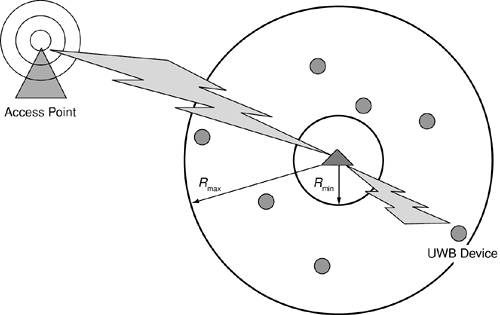If we now let Rmax tend to infinity, the victim receiver experiences a total interference power due to the UWB devices given by

Equation A-1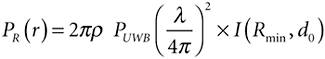where r is the UWB density in users/m2, PUWB is the transmission power for each UWB device, l is the wavelength, and I(Rmin, d0) is a factor depending on the environment through d0 and Rmin. Details of the model can be found in . From , the SINR at the victim receiver is defined by

Equation A-2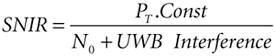N0 is the thermal noise (that is, we assume there is no neighboring channel interference), PT denotes the transmit power at the access point, Const is the path loss as a function of distance, and UWB Interference represents the total received power from all the UWB devices around the victim receiver. Thus in dBm, we get

Equation A-3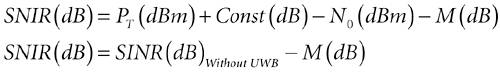Here we have defined M such that

Equation A-4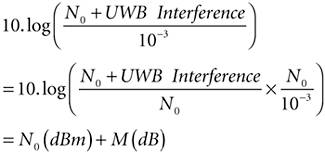Therefore, any increase of M dB due to UWB interference will result in an equal decrease in the SINR. Hence, if we assume that the system can support a degradation of M dB in SINR, we can deduce the total amount of UWB interference corresponding to an M dB degradation such that

Equation A-5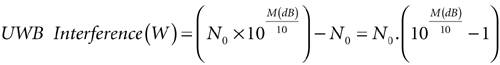We have also found a general value for UWB Interference as a function of the UWB transmitter density from Rmin to infinity such that

Equation A-6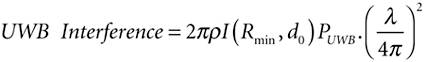with

Equation A-7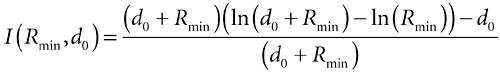and PUWB the UWB transmit power (fixed by the maximum of the emission mask). So, we can find the maximum permissible UWB transmitter density r under the constraint that the SINR decreases not more than M dB such that

Equation A-8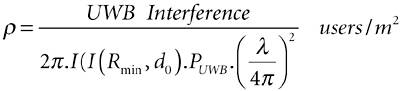Therefore, we can evaluate the permitted UWB density for different decreases in the SINR and estimate effects due to an introduction of UWB devices on IEEE 802.11a/b WLANs.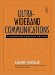Ultra-Wideband Communications: Fundamentals and Applications
ISBN: 0131463268
EAN: 2147483647
Year: 2005
Pages: 93

flylib.com © 2008-2017.
If you may any questions please contact us: flylib@qtcs.net